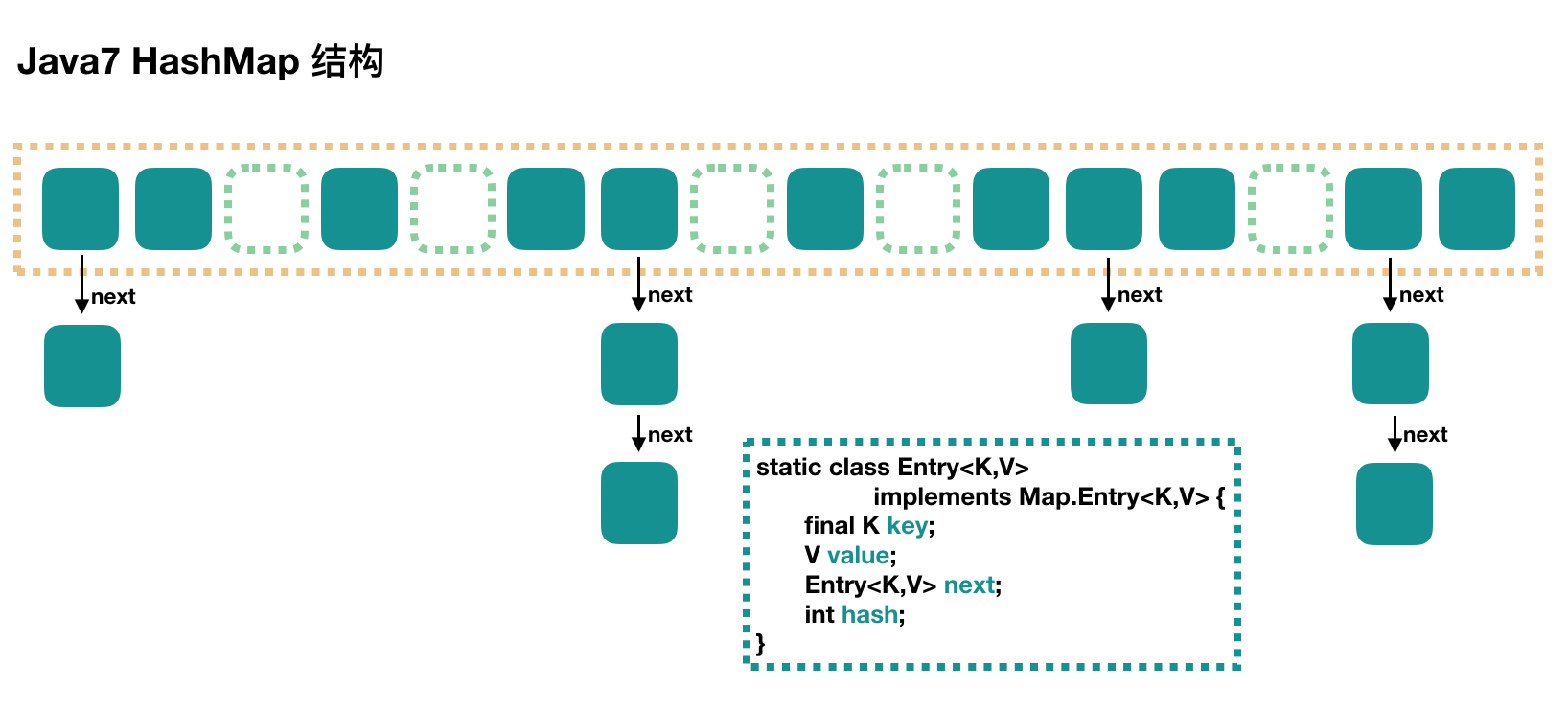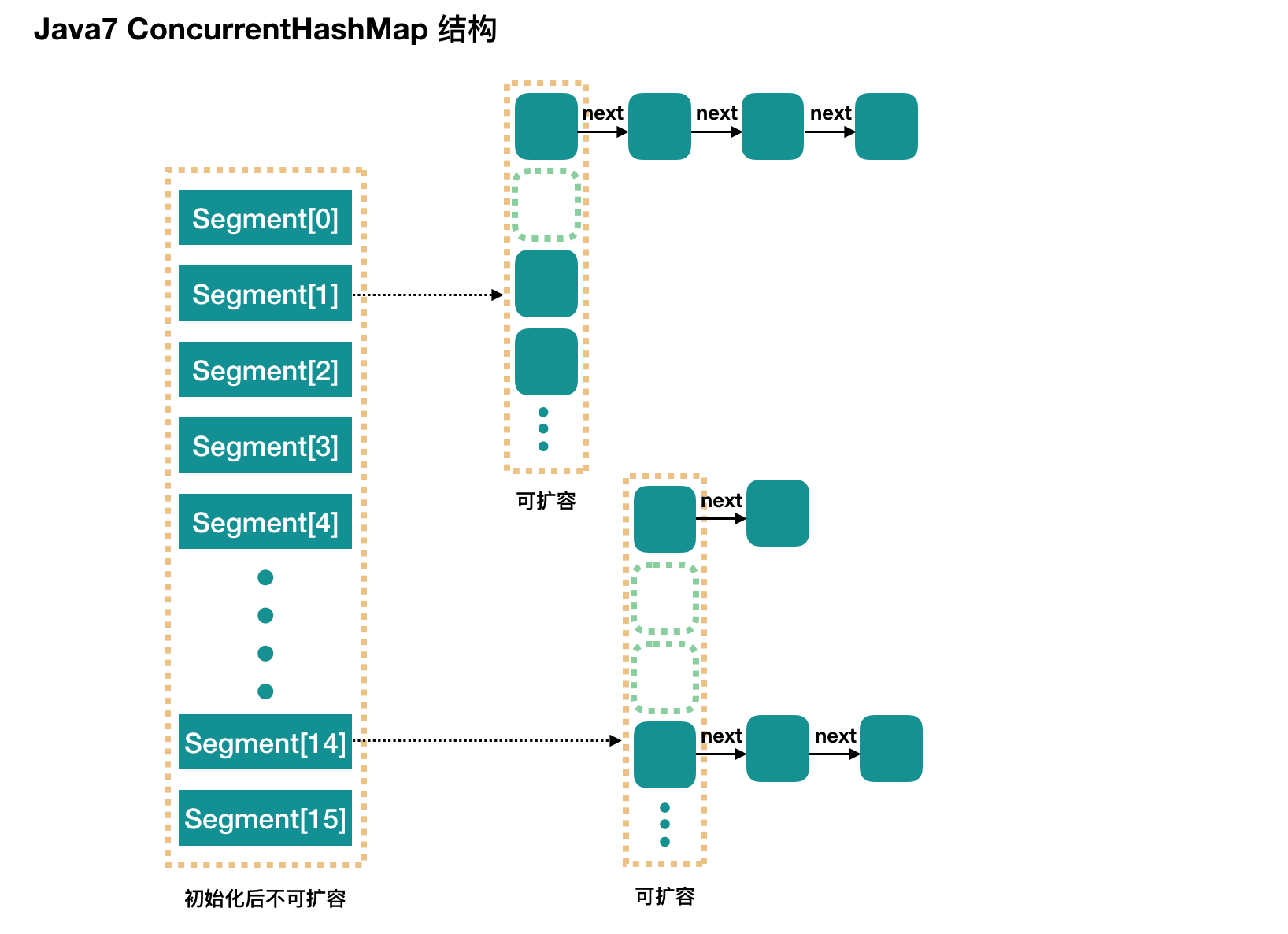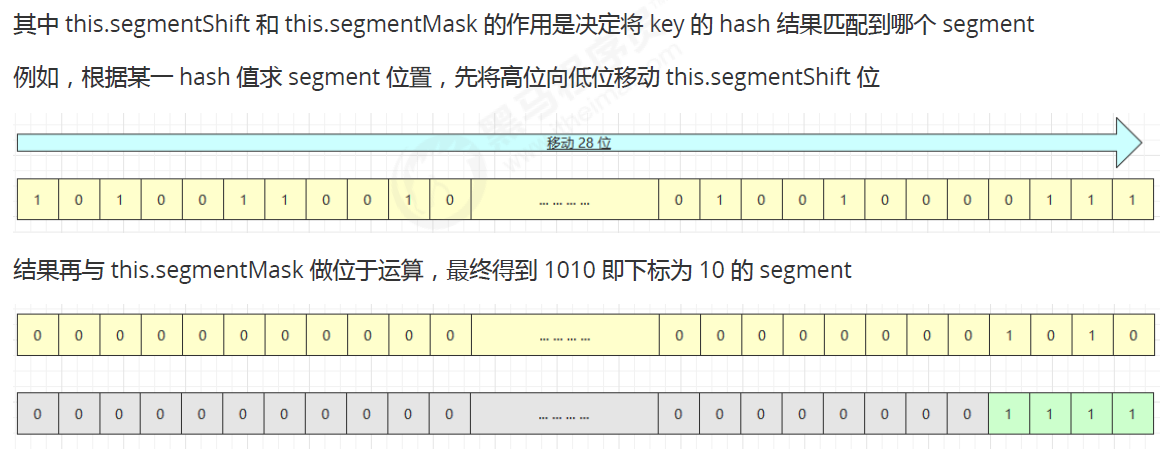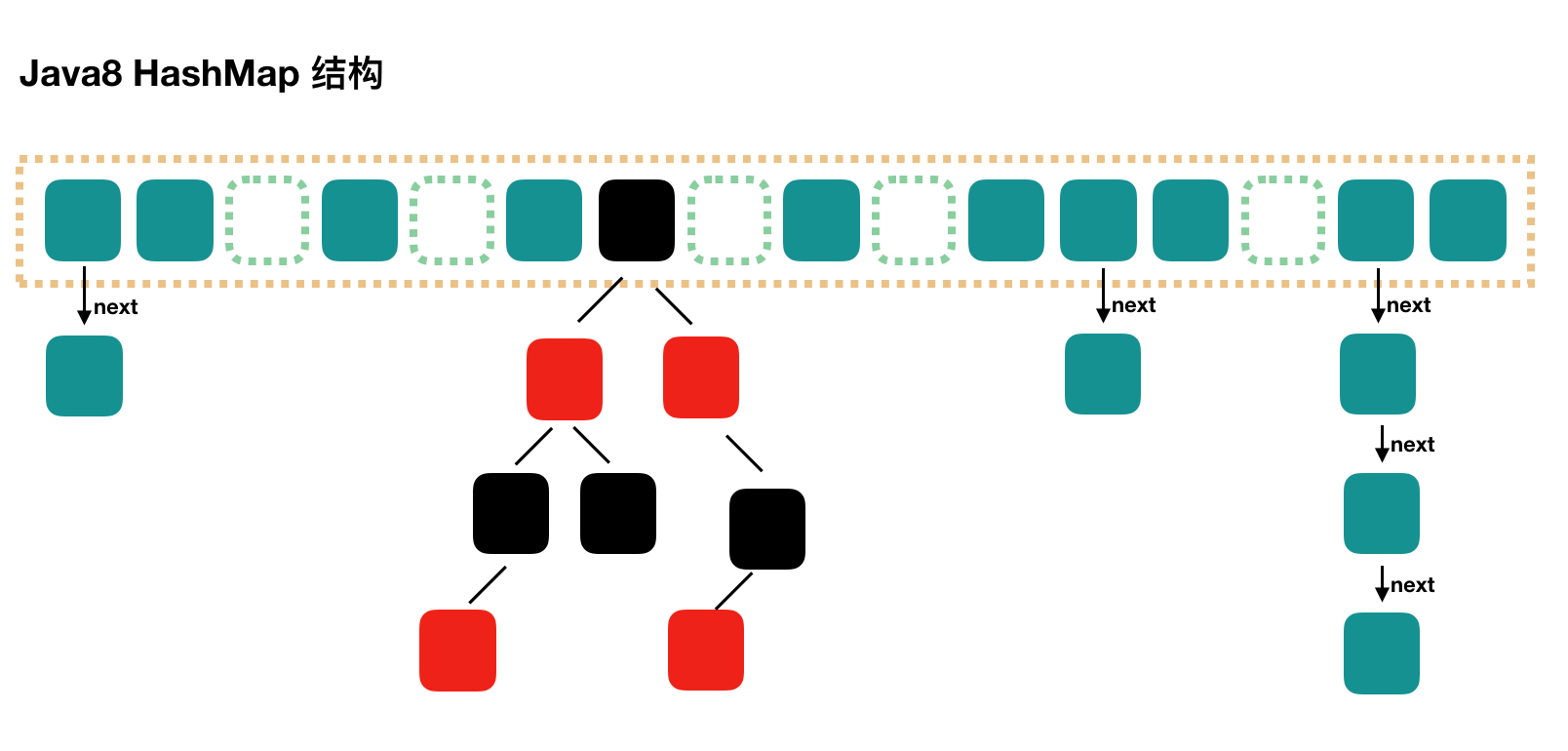# 1、Java7 HashMap

JDK 8 虽然将扩容算法做了调整，不再将元素加入链表头（而是保持与扩容前一样的顺序），但仍不意味着能够在多线程环境下能够安全扩容，还会出现其它问题（如扩容丢数据）。# 2、Java7 ConcurrentHashMappublic ConcurrentHashMap(int initialCapacity,
if (!(loadFactor > 0) || initialCapacity < 0 || concurrencyLevel <= 0)
throw new IllegalArgumentException();
if (concurrencyLevel > MAX_SEGMENTS)
concurrencyLevel = MAX_SEGMENTS;
// Find power-of-two sizes best matching arguments
int sshift = 0;
int ssize = 1;
// 计算并行级别 ssize，因为要保持并行级别是 2 的 n 次方
while (ssize < concurrencyLevel) {
++sshift;
ssize <<= 1;
}
// 我们这里先不要那么烧脑，用默认值，concurrencyLevel 为 16，sshift 为 4
// 那么计算出 segmentShift 为 28，segmentMask 为 15，后面会用到这两个值
this.segmentShift = 32 - sshift;

if (initialCapacity > MAXIMUM_CAPACITY)
initialCapacity = MAXIMUM_CAPACITY;

// initialCapacity 是设置整个 map 初始的大小，
// 这里根据 initialCapacity 计算 Segment 数组中每个位置可以分到的大小
// 如 initialCapacity 为 64，那么每个 Segment 或称之为"槽"可以分到 4 个
int c = initialCapacity / ssize;
if (c * ssize < initialCapacity)
++c;
// 默认 MIN_SEGMENT_TABLE_CAPACITY 是 2，这个值也是有讲究的，因为这样的话，对于具体的槽上，
// 插入一个元素不至于扩容，插入第二个的时候才会扩容
int cap = MIN_SEGMENT_TABLE_CAPACITY;
while (cap < c)
cap <<= 1;

// 创建 Segment 数组，
// 并创建数组的第一个元素 segment
Segment<K,V> s0 =
(HashEntry<K,V>[])new HashEntry[cap]);
Segment<K,V>[] ss = (Segment<K,V>[])new Segment[ssize];
// 往数组写入 segment
UNSAFE.putOrderedObject(ss, SBASE, s0); // ordered write of segments
this.segments = ss;
}



• Segment 数组长度为 16，不可以扩容
• Segment[i] 的默认大小为 2，负载因子是 0.75，得出初始阈值为1.5，也就是以后插入第一个元素不会触发扩容，插入第二个会进行第一次扩容
• 这里初始化了 segment，其他位置还是 null，至于为什么要初始化 segment，后面的代码会介绍
• 当前 segmentShift 的值为 32 - 4 =28，segmentMask 为 16 - 1 = 15，put流程

public V put(K key, V value) {
Segment<K,V> s;
if (value == null)
throw new NullPointerException();
// 1. 计算 key 的 hash 值
int hash = hash(key);
// 2. 根据 hash 值找到 Segment 数组中的位置 j
int j = (hash >>> segmentShift) & segmentMask;
// 刚刚说了，初始化的时候初始化了 segment，但是其他位置还是 null，
// ensureSegment(j) 对 segment[j] 进行初始化
if ((s = (Segment<K,V>)UNSAFE.getObject          // nonvolatile; recheck
(segments, (j << SSHIFT) + SBASE)) == null)
// 这时不能确定是否真的为 null, 因为其它线程也发现该 segment 为 null,
// 因此在 ensureSegment 里用 cas 方式保证该 segment 安全性
s = ensureSegment(j);
// 3. 插入新值到 槽 s 中
return s.put(key, hash, value, false);
}


segment 继承了可重入锁（ReentrantLock），它的 put 方法为

final V put(K key, int hash, V value, boolean onlyIfAbsent) {
// 在往该 segment 写入前，需要先获取该 segment 的独占锁
// 如果不成功, 进入 scanAndLockForPut 流程
// 如果是多核 cpu 最多 tryLock 64 次, 进入 lock 流程
// 在尝试期间, 还可以顺便看该节点在链表中有没有, 如果没有顺便创建出来
HashEntry<K,V> node = tryLock() ? null :
scanAndLockForPut(key, hash, value);

// 执行到这里 segment 已经被成功加锁, 可以安全执行
V oldValue;
try {
// 这个是 segment 内部的数组
HashEntry<K,V>[] tab = table;
// 再利用 hash 值，求应该放置的数组下标
int index = (tab.length - 1) & hash;
// first 是数组该位置处的链表的表头
HashEntry<K,V> first = entryAt(tab, index);

// 下面这串 for 循环虽然很长，不过也很好理解，想想该位置没有任何元素和已经存在一个链表这两种情况
for (HashEntry<K,V> e = first;;) {
if (e != null) {
K k;
if ((k = e.key) == key ||
(e.hash == hash && key.equals(k))) {
oldValue = e.value;
if (!onlyIfAbsent) {
// 覆盖旧值
e.value = value;
++modCount;
}
break;
}
// 继续顺着链表走
e = e.next;
}
else {

// // 1) 之前等待锁时, node 已经被创建, next 指向链表头
if (node != null)
node.setNext(first);
else
// 2) 创建新 node
node = new HashEntry<K,V>(hash, key, value, first);

int c = count + 1;
// 如果超过了该 segment 的阈值，这个 segment 需要扩容
if (c > threshold && tab.length < MAXIMUM_CAPACITY)
rehash(node); // 扩容后面也会具体分析
else
// 没有达到阈值，将 node 放到数组 tab 的 index 位置，
// 其实就是将新的节点设置成原链表的表头
setEntryAt(tab, index, node);
++modCount;
count = c;
oldValue = null;
break;
}
}
} finally {
// 解锁
unlock();
}
return oldValue;
}



ConcurrentHashMap 初始化的时候会初始化第一个槽 segment，对于其他槽来说，在插入第一个值的时候进行初始化。

private Segment<K,V> ensureSegment(int k) {
final Segment<K,V>[] ss = this.segments;
long u = (k << SSHIFT) + SBASE; // raw offset
Segment<K,V> seg;
if ((seg = (Segment<K,V>)UNSAFE.getObjectVolatile(ss, u)) == null) {
// 这里看到为什么之前要初始化 segment 了，
// 使用当前 segment 处的数组长度和负载因子来初始化 segment[k]
// 为什么要用“当前”，因为 segment 可能早就扩容过了
Segment<K,V> proto = ss;
int cap = proto.table.length;
int threshold = (int)(cap * lf);

// 初始化 segment[k] 内部的数组
HashEntry<K,V>[] tab = (HashEntry<K,V>[])new HashEntry[cap];
if ((seg = (Segment<K,V>)UNSAFE.getObjectVolatile(ss, u))
== null) { // 再次检查一遍该槽是否被其他线程初始化了。

Segment<K,V> s = new Segment<K,V>(lf, threshold, tab);
// 使用 while 循环，内部用 CAS，当前线程成功设值或其他线程成功设值后，退出
while ((seg = (Segment<K,V>)UNSAFE.getObjectVolatile(ss, u))
== null) {
if (UNSAFE.compareAndSwapObject(ss, u, null, seg = s))
break;
}
}
}
return seg;
}


private HashEntry<K,V> scanAndLockForPut(K key, int hash, V value) {
HashEntry<K,V> first = entryForHash(this, hash);
HashEntry<K,V> e = first;
HashEntry<K,V> node = null;
int retries = -1; // negative while locating node

// 循环获取锁
while (!tryLock()) {
HashEntry<K,V> f; // to recheck first below
if (retries < 0) {
if (e == null) {
if (node == null) // speculatively create node
// 进到这里说明数组该位置的链表是空的，没有任何元素
// 当然，进到这里的另一个原因是 tryLock() 失败，所以该槽存在并发，不一定是该位置
node = new HashEntry<K,V>(hash, key, value, null);
retries = 0;
}
else if (key.equals(e.key))
retries = 0;
else
// 顺着链表往下走
e = e.next;
}
// 重试次数如果超过 MAX_SCAN_RETRIES（单核1多核64），那么不抢了，进入到阻塞队列等待锁
//    lock() 是阻塞方法，直到获取锁后返回
else if (++retries > MAX_SCAN_RETRIES) {
lock();
break;
}
else if ((retries & 1) == 0 &&
// 这个时候是有大问题了，那就是有新的元素进到了链表，成为了新的表头
//     所以这边的策略是，相当于重新走一遍这个 scanAndLockForPut 方法
(f = entryForHash(this, hash)) != first) {
e = first = f; // re-traverse if entry changed
retries = -1;
}
}
return node;
}



// 方法参数上的 node 是这次扩容后，需要添加到新的数组中的数据。
private void rehash(HashEntry<K,V> node) {
HashEntry<K,V>[] oldTable = table;
int oldCapacity = oldTable.length;
// 2 倍
int newCapacity = oldCapacity << 1;
// 创建新数组
HashEntry<K,V>[] newTable =
(HashEntry<K,V>[]) new HashEntry[newCapacity];
// 新的掩码，如从 16 扩容到 32，那么 sizeMask 为 31，对应二进制 ‘000...00011111’
int sizeMask = newCapacity - 1;

// 遍历原数组，老套路，将原数组位置 i 处的链表拆分到 新数组位置 i 和 i+oldCap 两个位置
for (int i = 0; i < oldCapacity ; i++) {
// e 是链表的第一个元素
HashEntry<K,V> e = oldTable[i];
if (e != null) {
HashEntry<K,V> next = e.next;
// 计算应该放置在新数组中的位置，
// 假设原数组长度为 16，e 在 oldTable 处，那么 idx 只可能是 3 或者是 3 + 16 = 19
int idx = e.hash & sizeMask;
if (next == null)   // 该位置处只有一个元素，那比较好办
newTable[idx] = e;
else { // Reuse consecutive sequence at same slot
// e 是链表表头
HashEntry<K,V> lastRun = e;
// idx 是当前链表的头结点 e 的新位置
int lastIdx = idx;

// 下面这个 for 循环会找到一个 lastRun 节点，这个节点之后的所有元素是将要放到一起的
for (HashEntry<K,V> last = next;last != null;last = last.next) {
int k = last.hash & sizeMask;
if (k != lastIdx) {
lastIdx = k;
lastRun = last;
}
}
// 将 lastRun 及其之后的所有节点组成的这个链表放到 lastIdx 这个位置
newTable[lastIdx] = lastRun;
// 下面的操作是处理 lastRun 之前的节点，
//    这些节点可能分配在另一个链表中，也可能分配到上面的那个链表中
for (HashEntry<K,V> p = e; p != lastRun; p = p.next) {
V v = p.value;
int h = p.hash;
int k = h & sizeMask;
HashEntry<K,V> n = newTable[k];
newTable[k] = new HashEntry<K,V>(h, p.key, v, n);
}
}
}
}
// 将新来的 node 放到新数组中刚刚的 两个链表之一 的 头部
node.setNext(newTable[nodeIndex]);
newTable[nodeIndex] = node;
table = newTable;
}



get过程

• 计算 hash 值，找到 segment 数组中的具体位置，或我们前面用的“槽”
• 槽中也是一个数组，根据 hash 找到数组中具体的位置
• \到这里是链表了，顺着链表进行查找即可
public V get(Object key) {
Segment<K,V> s; // manually integrate access methods to reduce overhead
HashEntry<K,V>[] tab;
// 1\. hash 值
int h = hash(key);
long u = (((h >>> segmentShift) & segmentMask) << SSHIFT) + SBASE;
// 2\. 根据 hash 找到对应的 segment
if ((s = (Segment<K,V>)UNSAFE.getObjectVolatile(segments, u)) != null &&
(tab = s.table) != null) {
// 3\. 找到segment 内部数组相应位置的链表，遍历
for (HashEntry<K,V> e = (HashEntry<K,V>) UNSAFE.getObjectVolatile
(tab, ((long)(((tab.length - 1) & h)) << TSHIFT) + TBASE);
e != null; e = e.next) {
K k;
if ((k = e.key) == key || (e.hash == h && key.equals(k)))
return e.value;
}
}
return null;
}


## 并发问题分析

1、put 操作的线程安全性。

• 初始化槽，这个我们之前就说过了，使用了 CAS 来初始化 Segment 中的数组。
• 添加节点到链表的操作是插入到表头的，所以，如果这个时候 get 操作在链表遍历的过程已经到了中间，是不会影响的。当然，另一个并发问题就是get 操作在 put 之后，需要保证刚刚插入表头的节点被读取，这个依赖于 setEntryAt 方法中使用的
UNSAFE.putOrderedObject。
• 扩容。扩容是新创建了数组，然后进行迁移数据，最后面将 newTable 设置给属性table。所以，如果 get 操作此时也在进行，那么也没关系，如果 get 先行，那么就是在旧的 table 上做查询操作；而 put先行，那么 put 操作的可见性保证就是 table 使用了 volatile 关键字。

2、remove 操作的线程安全性。

• remove 操作我们没有分析源码，所以这里说的读者感兴趣的话还是需要到源码中去求实一下的。
• get 操作需要遍历链表，但是 remove 操作会"破坏"链表。
• 如果 remove 破坏的节点 get 操作已经过去了，那么这里不存在任何问题。
• 如果 remove 先破坏了一个节点，分两种情况考虑。 1、如果此节点是头结点，那么需要将头结点的 next 设置为数组该位置的元素，table 虽然使用了 volatile 修饰，但是 volatile 并不能提供数组内部操作的可见性保证，所以源码中使用了 UNSAFE 来操作数组，请看方法 setEntryAt。2、如果要删除的节点不是头结点，它会将要删除节点的后继节点接到前驱节点中，这里的并发保证就是 next 属性是 volatile 的。

# 3、Java8 HashMap

Java8 对 HashMap 进行了一些修改，最大的不同就是利用了红黑树，所以其由 数组+链表+红黑树 组成。Java7 中使用 Entry 来代表每个 HashMap 中的数据节点，Java8 中使用 Node，基本没有区别，都是 key，value，hash 和 next 这四个属性，不过，Node 只能用于链表的情况，红黑树的情况需要使用 TreeNode。

# 4、Java8 ConcurrentHashMap

// 这构造函数里，什么都不干
public ConcurrentHashMap() {
}
public ConcurrentHashMap(int initialCapacity) {
if (initialCapacity < 0)
throw new IllegalArgumentException();
int cap = ((initialCapacity >= (MAXIMUM_CAPACITY >>> 1)) ?
MAXIMUM_CAPACITY :
tableSizeFor(initialCapacity + (initialCapacity >>> 1) + 1));
this.sizeCtl = cap;
}


put 过程分析

public V put(K key, V value) {
return putVal(key, value, false);
}
final V putVal(K key, V value, boolean onlyIfAbsent) {
if (key == null || value == null) throw new NullPointerException();
// 得到 hash 值
// 用于记录相应链表的长度
int binCount = 0;
for (Node<K,V>[] tab = table;;) {
Node<K,V> f; int n, i, fh;
// 如果数组"空"，进行数组初始化
if (tab == null || (n = tab.length) == 0)
// 初始化数组，后面会详细介绍
tab = initTable();

// 找该 hash 值对应的数组下标，得到第一个节点 f
else if ((f = tabAt(tab, i = (n - 1) & hash)) == null) {
// 如果数组该位置为空，
//    用一次 CAS 操作将这个新值放入其中即可，这个 put 操作差不多就结束了，可以拉到最后面了
//          如果 CAS 失败，那就是有并发操作，进到下一个循环就好了
if (casTabAt(tab, i, null,
new Node<K,V>(hash, key, value, null)))
break;                   // no lock when adding to empty bin
}
// hash 居然可以等于 MOVED，这个需要到后面才能看明白，不过从名字上也能猜到，肯定是因为在扩容
else if ((fh = f.hash) == MOVED)
// 帮助数据迁移，这个等到看完数据迁移部分的介绍后，再理解这个就很简单了
tab = helpTransfer(tab, f);

else { // 到这里就是说，f 是该位置的头结点，而且不为空

V oldVal = null;
// 获取数组该位置的头结点的监视器锁
synchronized (f) {
if (tabAt(tab, i) == f) {
if (fh >= 0) { // 头结点的 hash 值大于 0，说明是链表
// 用于累加，记录链表的长度
binCount = 1;
// 遍历链表
for (Node<K,V> e = f;; ++binCount) {
K ek;
// 如果发现了"相等"的 key，判断是否要进行值覆盖，然后也就可以 break 了
if (e.hash == hash &&
((ek = e.key) == key ||
(ek != null && key.equals(ek)))) {
oldVal = e.val;
if (!onlyIfAbsent)
e.val = value;
break;
}
// 到了链表的最末端，将这个新值放到链表的最后面
Node<K,V> pred = e;
if ((e = e.next) == null) {
pred.next = new Node<K,V>(hash, key,
value, null);
break;
}
}
}
else if (f instanceof TreeBin) { // 红黑树
Node<K,V> p;
binCount = 2;
// 调用红黑树的插值方法插入新节点
if ((p = ((TreeBin<K,V>)f).putTreeVal(hash, key,
value)) != null) {
oldVal = p.val;
if (!onlyIfAbsent)
p.val = value;
}
}
}
}

if (binCount != 0) {
// 判断是否要将链表转换为红黑树，临界值和 HashMap 一样，也是 8
if (binCount >= TREEIFY_THRESHOLD)
// 这个方法和 HashMap 中稍微有一点点不同，那就是它不是一定会进行红黑树转换，
// 如果当前数组的长度小于 64，那么会选择进行数组扩容，而不是转换为红黑树
//    具体源码我们就不看了，扩容部分后面说
treeifyBin(tab, i);
if (oldVal != null)
return oldVal;
break;
}
}
}
//
return null;
}


put 的主流程看完了，但是至少留下了几个问题，第一个是初始化，第二个是扩容，第三个是帮助数据迁移，这些我们都会在后面进行一一介绍。

private final Node<K,V>[] initTable() {
Node<K,V>[] tab; int sc;
while ((tab = table) == null || tab.length == 0) {
// 初始化的"功劳"被其他线程"抢去"了
if ((sc = sizeCtl) < 0)
Thread.yield(); // lost initialization race; just spin
// CAS 一下，将 sizeCtl 设置为 -1，代表抢到了锁
else if (U.compareAndSwapInt(this, SIZECTL, sc, -1)) {
try {
if ((tab = table) == null || tab.length == 0) {
// DEFAULT_CAPACITY 默认初始容量是 16
int n = (sc > 0) ? sc : DEFAULT_CAPACITY;
// 初始化数组，长度为 16 或初始化时提供的长度
Node<K,V>[] nt = (Node<K,V>[])new Node<?,?>[n];
// 将这个数组赋值给 table，table 是 volatile 的
table = tab = nt;
// 如果 n 为 16 的话，那么这里 sc = 12
// 其实就是 0.75 * n
sc = n - (n >>> 2);
}
} finally {
// 设置 sizeCtl 为 sc，我们就当是 12 吧
sizeCtl = sc;
}
break;
}
}
return tab;
}


private final void treeifyBin(Node<K,V>[] tab, int index) {
Node<K,V> b; int n, sc;
if (tab != null) {
// MIN_TREEIFY_CAPACITY 为 64
// 所以，如果数组长度小于 64 的时候，其实也就是 32 或者 16 或者更小的时候，会进行数组扩容
if ((n = tab.length) < MIN_TREEIFY_CAPACITY)
// 后面我们再详细分析这个方法
tryPresize(n << 1);
// b 是头结点
else if ((b = tabAt(tab, index)) != null && b.hash >= 0) {
// 加锁
synchronized (b) {

if (tabAt(tab, index) == b) {
// 下面就是遍历链表，建立一颗红黑树
TreeNode<K,V> hd = null, tl = null;
for (Node<K,V> e = b; e != null; e = e.next) {
TreeNode<K,V> p =
new TreeNode<K,V>(e.hash, e.key, e.val,
null, null);
if ((p.prev = tl) == null)
hd = p;
else
tl.next = p;
tl = p;
}
// 将红黑树设置到数组相应位置中
setTabAt(tab, index, new TreeBin<K,V>(hd));
}
}
}
}
}


// 首先要说明的是，方法参数 size 传进来的时候就已经翻了倍了
private final void tryPresize(int size) {
// c：size 的 1.5 倍，再加 1，再往上取最近的 2 的 n 次方。
int c = (size >= (MAXIMUM_CAPACITY >>> 1)) ? MAXIMUM_CAPACITY :
tableSizeFor(size + (size >>> 1) + 1);
int sc;
while ((sc = sizeCtl) >= 0) {
Node<K,V>[] tab = table; int n;

// 这个 if 分支和之前说的初始化数组的代码基本上是一样的，在这里，我们可以不用管这块代码
if (tab == null || (n = tab.length) == 0) {
n = (sc > c) ? sc : c;
if (U.compareAndSwapInt(this, SIZECTL, sc, -1)) {
try {
if (table == tab) {
@SuppressWarnings("unchecked")
Node<K,V>[] nt = (Node<K,V>[])new Node<?,?>[n];
table = nt;
sc = n - (n >>> 2); // 0.75 * n
}
} finally {
sizeCtl = sc;
}
}
}
else if (c <= sc || n >= MAXIMUM_CAPACITY)
break;
else if (tab == table) {
// 我没看懂 rs 的真正含义是什么，不过也关系不大
int rs = resizeStamp(n);

if (sc < 0) {
Node<K,V>[] nt;
if ((sc >>> RESIZE_STAMP_SHIFT) != rs || sc == rs + 1 ||
sc == rs + MAX_RESIZERS || (nt = nextTable) == null ||
transferIndex <= 0)
break;
// 2\. 用 CAS 将 sizeCtl 加 1，然后执行 transfer 方法
//    此时 nextTab 不为 null
if (U.compareAndSwapInt(this, SIZECTL, sc, sc + 1))
transfer(tab, nt);
}
// 1\. 将 sizeCtl 设置为 (rs << RESIZE_STAMP_SHIFT) + 2)
//     我是没看懂这个值真正的意义是什么？不过可以计算出来的是，结果是一个比较大的负数
//  调用 transfer 方法，此时 nextTab 参数为 null
else if (U.compareAndSwapInt(this, SIZECTL, sc,
(rs << RESIZE_STAMP_SHIFT) + 2))
transfer(tab, null);
}
}
}


• 虽然我们之前说的 tryPresize 方法中多次调用 transfer 不涉及多线程，但是这个 transfer 方法可以在其他地方被调用，典型地，我们之前在说 put 方法的时候就说过了，请往上看 put 方法，是不是有个地方调用了 helpTransfer 方法，helpTransfer 方法会调用 transfer 方法的。
• 此方法支持多线程执行，外围调用此方法的时候，会保证第一个发起数据迁移的线程，nextTab 参数为 null，之后再调用此方法的时候，nextTab 不会为 null。
• 阅读源码之前，先要理解并发操作的机制。原数组长度为 n，所以我们有 n 个迁移任务，让每个线程每次负责一个小任务是最简单的，每做完一个任务再检测是否有其他没做完的任务，帮助迁移就可以了，而 Doug Lea 使用了一个 stride，简单理解就是步长，每个线程每次负责迁移其中的一部分，如每次迁移 16 个小任务。所以，我们就需要一个全局的调度者来安排哪个线程执行哪几个任务，这个就是属性 transferIndex 的作用。
第一个发起数据迁移的线程会将 transferIndex 指向原数组最后的位置，然后从后往前的 stride 个任务属于第一个线程，然后将 transferIndex 指向新的位置，再往前的 stride 个任务属于第二个线程，依此类推。当然，这里说的第二个线程不是真的一定指代了第二个线程，也可以是同一个线程，这个读者应该能理解吧。其实就是将一个大的迁移任务分为了一个个任务包。
private final void transfer(Node<K,V>[] tab, Node<K,V>[] nextTab) {
int n = tab.length, stride;

// stride 在单核下直接等于 n，多核模式下为 (n>>>3)/NCPU，最小值是 16
// stride 可以理解为”步长“，有 n 个位置是需要进行迁移的，
//   将这 n 个任务分为多个任务包，每个任务包有 stride 个任务
if ((stride = (NCPU > 1) ? (n >>> 3) / NCPU : n) < MIN_TRANSFER_STRIDE)
stride = MIN_TRANSFER_STRIDE; // subdivide range

// 如果 nextTab 为 null，先进行一次初始化
//    前面我们说了，外围会保证第一个发起迁移的线程调用此方法时，参数 nextTab 为 null
//       之后参与迁移的线程调用此方法时，nextTab 不会为 null
if (nextTab == null) {
try {
// 容量翻倍
Node<K,V>[] nt = (Node<K,V>[])new Node<?,?>[n << 1];
nextTab = nt;
} catch (Throwable ex) {      // try to cope with OOME
sizeCtl = Integer.MAX_VALUE;
return;
}
// nextTable 是 ConcurrentHashMap 中的属性
nextTable = nextTab;
// transferIndex 也是 ConcurrentHashMap 的属性，用于控制迁移的位置
transferIndex = n;
}

int nextn = nextTab.length;

// ForwardingNode 翻译过来就是正在被迁移的 Node
// 这个构造方法会生成一个Node，key、value 和 next 都为 null，关键是 hash 为 MOVED
// 后面我们会看到，原数组中位置 i 处的节点完成迁移工作后，
//    就会将位置 i 处设置为这个 ForwardingNode，用来告诉其他线程该位置已经处理过了
//    所以它其实相当于是一个标志。
ForwardingNode<K,V> fwd = new ForwardingNode<K,V>(nextTab);

boolean finishing = false; // to ensure sweep before committing nextTab

/*
* 下面这个 for 循环，最难理解的在前面，而要看懂它们，应该先看懂后面的，然后再倒回来看
*
*/

// i 是位置索引，bound 是边界，注意是从后往前
for (int i = 0, bound = 0;;) {
Node<K,V> f; int fh;

// 下面这个 while 真的是不好理解
//   简单理解结局：i 指向了 transferIndex，bound 指向了 transferIndex-stride
int nextIndex, nextBound;
if (--i >= bound || finishing)

// 将 transferIndex 值赋给 nextIndex
// 这里 transferIndex 一旦小于等于 0，说明原数组的所有位置都有相应的线程去处理了
else if ((nextIndex = transferIndex) <= 0) {
i = -1;
}
else if (U.compareAndSwapInt
(this, TRANSFERINDEX, nextIndex,
nextBound = (nextIndex > stride ?
nextIndex - stride : 0))) {
// 看括号中的代码，nextBound 是这次迁移任务的边界，注意，是从后往前
bound = nextBound;
i = nextIndex - 1;
}
}
if (i < 0 || i >= n || i + n >= nextn) {
int sc;
if (finishing) {
// 所有的迁移操作已经完成
nextTable = null;
// 将新的 nextTab 赋值给 table 属性，完成迁移
table = nextTab;
// 重新计算 sizeCtl：n 是原数组长度，所以 sizeCtl 得出的值将是新数组长度的 0.75 倍
sizeCtl = (n << 1) - (n >>> 1);
return;
}

// 之前我们说过，sizeCtl 在迁移前会设置为 (rs << RESIZE_STAMP_SHIFT) + 2
// 然后，每有一个线程参与迁移就会将 sizeCtl 加 1，
// 这里使用 CAS 操作对 sizeCtl 进行减 1，代表做完了属于自己的任务
if (U.compareAndSwapInt(this, SIZECTL, sc = sizeCtl, sc - 1)) {
// 任务结束，方法退出
if ((sc - 2) != resizeStamp(n) << RESIZE_STAMP_SHIFT)
return;

// 到这里，说明 (sc - 2) == resizeStamp(n) << RESIZE_STAMP_SHIFT，
// 也就是说，所有的迁移任务都做完了，也就会进入到上面的 if(finishing){} 分支了
i = n; // recheck before commit
}
}
// 如果位置 i 处是空的，没有任何节点，那么放入刚刚初始化的 ForwardingNode ”空节点“
else if ((f = tabAt(tab, i)) == null)
advance = casTabAt(tab, i, null, fwd);
// 该位置处是一个 ForwardingNode，代表该位置已经迁移过了
else if ((fh = f.hash) == MOVED)
else {
// 对数组该位置处的结点加锁，开始处理数组该位置处的迁移工作
synchronized (f) {
if (tabAt(tab, i) == f) {
Node<K,V> ln, hn;
// 头结点的 hash 大于 0，说明是链表的 Node 节点
if (fh >= 0) {
// 下面这一块和 Java7 中的 ConcurrentHashMap 迁移是差不多的，
// 需要将链表一分为二，
//   找到原链表中的 lastRun，然后 lastRun 及其之后的节点是一起进行迁移的
//   lastRun 之前的节点需要进行克隆，然后分到两个链表中
int runBit = fh & n;
Node<K,V> lastRun = f;
for (Node<K,V> p = f.next; p != null; p = p.next) {
int b = p.hash & n;
if (b != runBit) {
runBit = b;
lastRun = p;
}
}
if (runBit == 0) {
ln = lastRun;
hn = null;
}
else {
hn = lastRun;
ln = null;
}
for (Node<K,V> p = f; p != lastRun; p = p.next) {
int ph = p.hash; K pk = p.key; V pv = p.val;
if ((ph & n) == 0)
ln = new Node<K,V>(ph, pk, pv, ln);
else
hn = new Node<K,V>(ph, pk, pv, hn);
}
// 其中的一个链表放在新数组的位置 i
setTabAt(nextTab, i, ln);
// 另一个链表放在新数组的位置 i+n
setTabAt(nextTab, i + n, hn);
// 将原数组该位置处设置为 fwd，代表该位置已经处理完毕，
//    其他线程一旦看到该位置的 hash 值为 MOVED，就不会进行迁移了
setTabAt(tab, i, fwd);
}
else if (f instanceof TreeBin) {
// 红黑树的迁移
TreeBin<K,V> t = (TreeBin<K,V>)f;
TreeNode<K,V> lo = null, loTail = null;
TreeNode<K,V> hi = null, hiTail = null;
int lc = 0, hc = 0;
for (Node<K,V> e = t.first; e != null; e = e.next) {
int h = e.hash;
TreeNode<K,V> p = new TreeNode<K,V>
(h, e.key, e.val, null, null);
if ((h & n) == 0) {
if ((p.prev = loTail) == null)
lo = p;
else
loTail.next = p;
loTail = p;
++lc;
}
else {
if ((p.prev = hiTail) == null)
hi = p;
else
hiTail.next = p;
hiTail = p;
++hc;
}
}
// 如果一分为二后，节点数少于 8，那么将红黑树转换回链表
ln = (lc <= UNTREEIFY_THRESHOLD) ? untreeify(lo) :
(hc != 0) ? new TreeBin<K,V>(lo) : t;
hn = (hc <= UNTREEIFY_THRESHOLD) ? untreeify(hi) :
(lc != 0) ? new TreeBin<K,V>(hi) : t;

// 将 ln 放置在新数组的位置 i
setTabAt(nextTab, i, ln);
// 将 hn 放置在新数组的位置 i+n
setTabAt(nextTab, i + n, hn);
// 将原数组该位置处设置为 fwd，代表该位置已经处理完毕，
//    其他线程一旦看到该位置的 hash 值为 MOVED，就不会进行迁移了
setTabAt(tab, i, fwd);
}
}
}
}
}
}


get 过程分析

• 计算 hash 值
• 根据 hash 值找到数组对应位置: (n - 1) & h
• 根据该位置处结点性质进行相应查找
• 如果该位置为 null，那么直接返回 null 就可以了
• 如果该位置处的节点刚好就是我们需要的，返回该节点的值即可
• 如果该位置节点的 hash 值小于 0，说明正在扩容，或者是红黑树，后面我们再介绍 find 方法
• 如果以上 3 条都不满足，那就是链表，进行遍历比对即可
public V get(Object key) {
Node<K,V>[] tab; Node<K,V> e, p; int n, eh; K ek;
if ((tab = table) != null && (n = tab.length) > 0 &&
(e = tabAt(tab, (n - 1) & h)) != null) {
// 判断头结点是否就是我们需要的节点
if ((eh = e.hash) == h) {
if ((ek = e.key) == key || (ek != null && key.equals(ek)))
return e.val;
}
// 如果头结点的 hash 小于 0，说明 正在扩容，或者该位置是红黑树
else if (eh < 0)
// 参考 ForwardingNode.find(int h, Object k) 和 TreeBin.find(int h, Object k)
return (p = e.find(h, key)) != null ? p.val : null;

// 遍历链表
while ((e = e.next) != null) {
if (e.hash == h &&
((ek = e.key) == key || (ek != null && key.equals(ek))))
return e.val;
}
}
return null;
}

12-3010万+04-1052
04-1297
07-1664
03-2450
05-16931
07-26197
03-316599
12-011073
03-1165